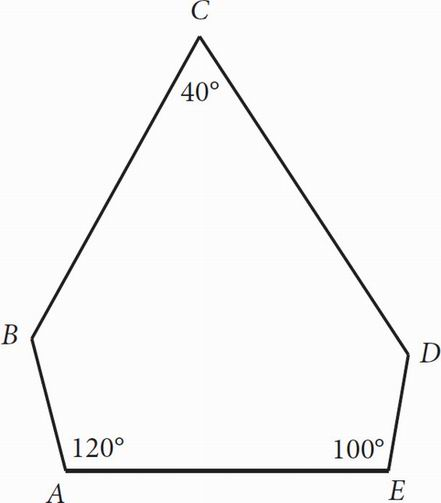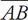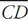# SAT Math Multiple Choice Question 162: Answer and Explanation

### Test Information

Question: 162

12.Note: Figure not drawn to scale.

In the figure above, ∠ABC ≅ ∠CDE. Which of the following is true?

• A. AB || CD
• B. BC || AE
• C. CD || AE
• D. BCAE

A Label the congruent angles, ∠ABC and ∠CDE, as such. In order to find the measure of those angles, use the formula 180(n - 2), where n is the number of sides, to determine the sum of the interior angles of the figure. Because the figure has five sides, plug 5 in for n to get 180(5 - 2), or 180(3), which equals 540. Subtract 120 to get 420. Subtract 100 to get 320. Subtract 40 to get 280. Since the two remaining angles are congruent, divide by 2 to find that the two unlabeled angles are both equal to 140. Because ∠ABC and ∠BCD have a combined measure of 180,andare parallel. Therefore, (A) accurately describes the relationships in the figure.